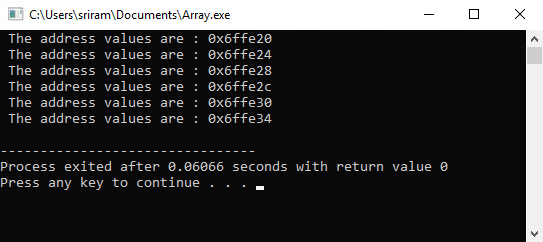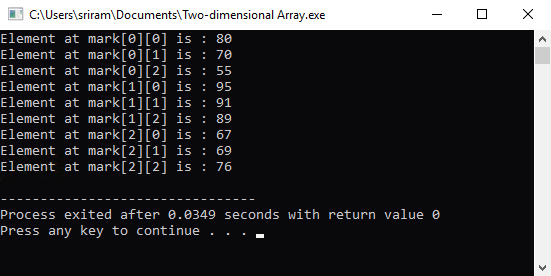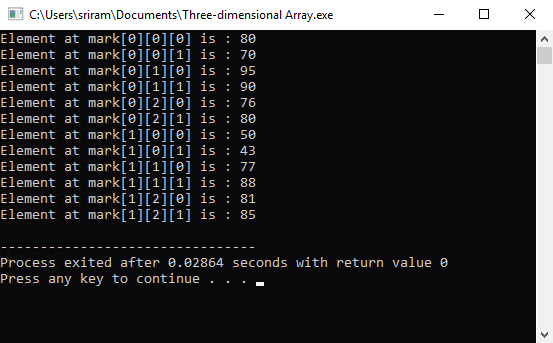# Understanding Arrays in C++

22 Aug 2022
Intermediate
4.14K Views

Array is a data structure in C++ programming language which can be used to store large amounts of data of the same type. The general definition of an array is “An array is a group of elements of the same name and the same datatype.”

### Syntax of declaring and initializing arrays

In C++, you can declare an array with the following syntax :

```
Datatype array_name[array_size];
```

The syntax to initialize an array is ,

``` Datatype array_name[array_size]={value1, value2, value3 …. valuen};
```

You need to remember that you cannot create more values within the braces than the value declared in the array_size.

## Need for arrays in C++ Programming

To understand why you must use an array, let’s take the below example

``` int a = 1;
int b = 2;
int c = 3;
…
…
…
…
int xyz = 10;
```

Instead of declaring the variables separately, and then performing operations individually on each of these variables, you can define them together in an array.

``` int arr={1, 2, 3, …. , 10};
```
arr
arr
arr
arr
arr
arr
arr
arr
arr
arr

These types of arrays are referred to as single dimensional arrays. In this case, the compiler will assign contiguous memory locations to the variables starting from arr till arr. The array index starts from 0 and ranges to n-1, where n is the last value defined in the array. You can also define the array as arr[] in which case an array with the memory just as big to hold the variables will be created.

## Types of Arrays

In C++, there are two types of arrays :

• One dimensional array (or) Single dimensional array

• Multi-dimensional array

### One dimensional array

In a 1-D array, the array elements are arranged in a linear fashion in a single dimension. The syntax of a 1-D array is :

``` Datatype array_name [array_size];
```
Example of a single dimensional array
``` #include <iostream>
using namespace std;

int main()
{
int a;
for (int i=0; i<=5; i++)
cout <<" The address values are : " << &a[i] << '\n';
}
```

The output of this program will display the address values of a,a,a,a & a :### Multi-dimensional array

Multi-dimensional array is nothing but a matrix of values. It’s an array or arrays (two-dimensional) or array of array of arrays (three-dimensional).

#### Two-dimensional array

For example, we can consider a two-dimensional array (mark) that stores the marks of 3 subjects of 3 different students. The values will be stored in the following manner :

Mark
Mark
Mark
Mark
Mark
Mark
Mark
Mark
Mark

The syntax to define a multi-dimensional array is :

``` datatype array_name[array_row_size][[array_column_size]
```

Example : int mark = {{80,70,55}, {95,91,89}, {67,69,76}};

Example program for two-dimensional array :

``` #include <iostream>
using namespace std;

int main()
{
}int mark = {{80,70,55}, {95,91,89}, {67,69,76}};
for (int row = 0; row <= 2; row++)
{
for (int column = 0; column <= 2; column++)
{
cout << "Element at mark[" << row << "][" << column << "] is : " << mark[row][column] << '\n';
}
}
}
```

The output of this program will be :#### Three-dimensional array

A three-dimensional array is the same as a two-dimensional array, just that there is one more dimension added to the array. In the previous example, we saw that there were two loop statements to traverse through the array elements. In this case, we will have three loop statements to traverse through the array elements.

Example of a three-dimensional array declaration
``` int array = {{80,70,55,64}, {95,91,89,90},
{67,69,76,80},{50,55,43,61},{77,88,67,98},{81,85,89,79}};
```
Example program for three-dimensional array
``` #include <iostream>
using namespace std;

int main()
{
int array = { { {80,70}, {95,90}, {76,80} }, { {50,43}, {77,88}, {81,85} } };
for (int a = 0; a < 2; a++)
{
for (int b = 0; b < 3; b++)
{
for (int c = 0; c < 2; c++)
cout << "Element at mark[" << a << "][" << b << "][" << c << "] is : " << array[a][b][c] << '\n';
}
}
}
```

The output of this program will be##### Summary

In this chapter, we have taken a look at the following topics :

• Introduction to arrays in C++ programming

• Why do we need to use arrays?

• Types of arrays